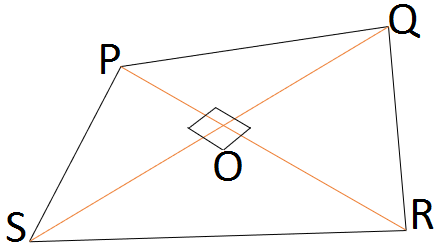Question of The Day26-10-2020

Three sides of a quadrilateral PQRS are PQ = 11 cm, QR = 9 cm and RS = 12 cm. If diagonal PR and QS intersect each other at 900, then what will be the length of side SP?

Correct Answer : d ) 7.6

Explanation :

Depicting the given informationIn ∆ POQ

Using Pythagoras theorem

PQ2 = OP2 + OQ2 ……. (1)

In ∆ QOR

Using Pythagoras theorem

QR2 = OQ2 + OR2 ……. (2)

In ∆ ROS

Using Pythagoras theorem

RS2 = OR2 + OS2 ……. (3)

In ∆ POS

Using Pythagoras theorem

PS2 = OP2 + OS2 ……. (4)

Adding equation 1, 2, 3 and 4

PQ2 + QR2 + RS2 + PS2 = 2 (OP2 + OQ2 + OR2 + OS2)

From equation 1 and 3

⇒ PQ2 + QR2 + RS2 + PS2 = 2 (PQ2 + RS2)

⇒ PQ2 + RS2 = QR2 + PS2

Now, putting the value given in question

112 + 92 = 122 + SP2

⇒ SP2 = 121 + 81 – 144 = 58

⇒SP=$$\sqrt58$$ ~ 7.6

Hence, (d) is the correct answer.

Such type of question is asked in various government exams like SSC CGL, SSC MTS, SSC CPO, SSC CHSL, RRB JE, RRB NTPC, RRB GROUP D, RRB OFFICER SCALE-I, IBPS PO, IBPS SO, RRB Office Assistant, IBPS Clerk, RBI Assistant, IBPS RRB OFFICER SCALE 2&3, UPSC CDS, UPSC NDA etc.

Read Daily Current Affairs, Banking Awareness, Hindi Current Affairs, Word of the Day, and attempt free mock tests at PendulumEdu and boost your preparation for the actual exam.0